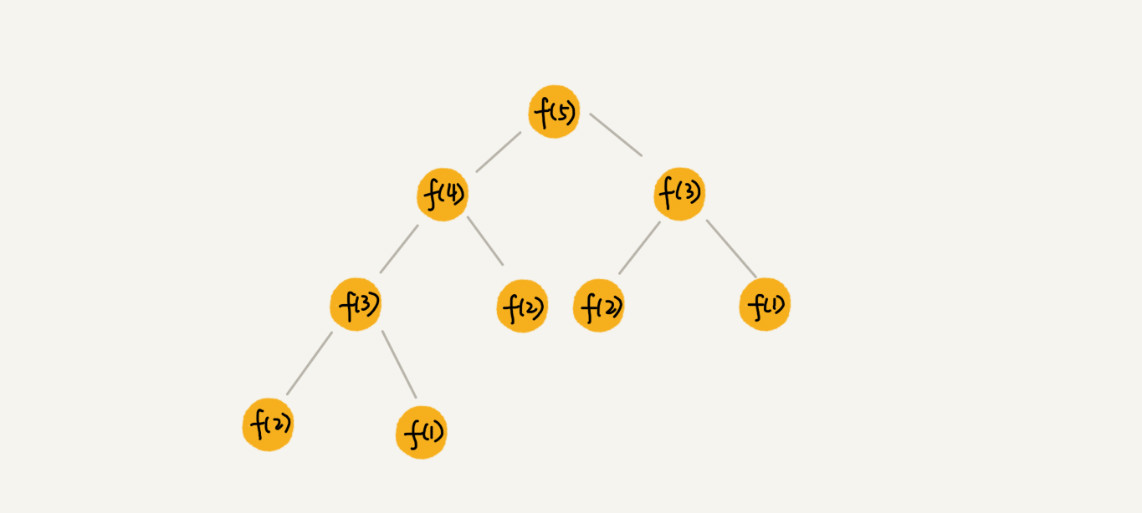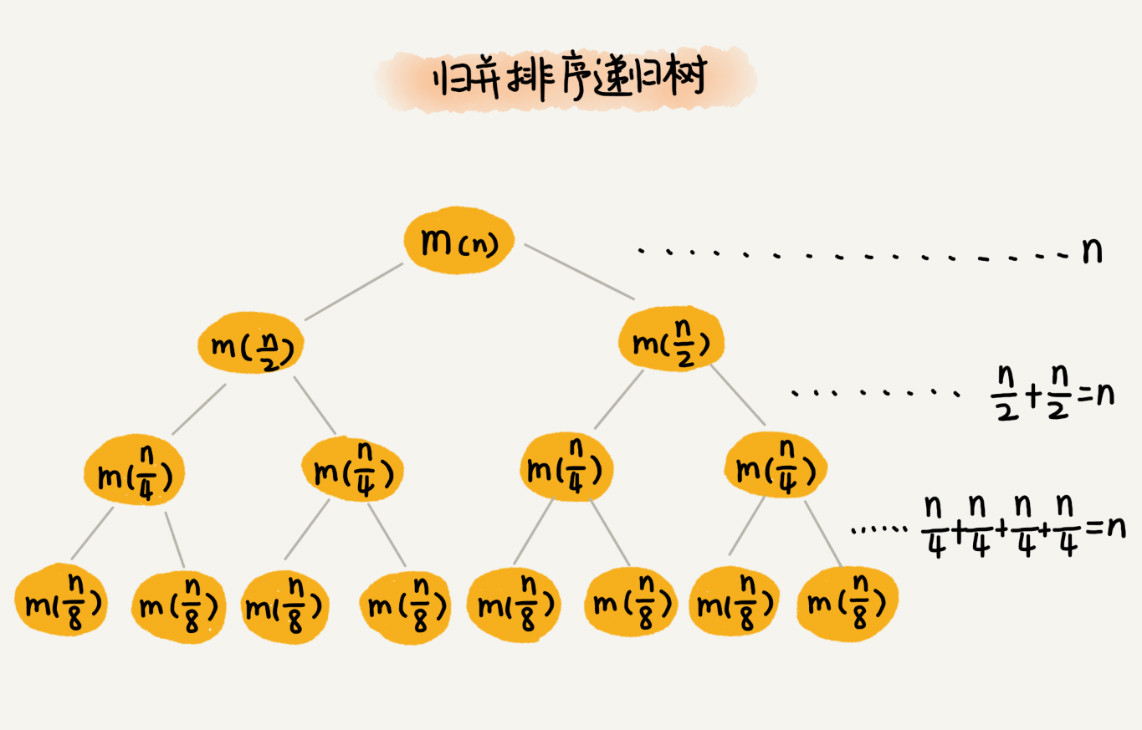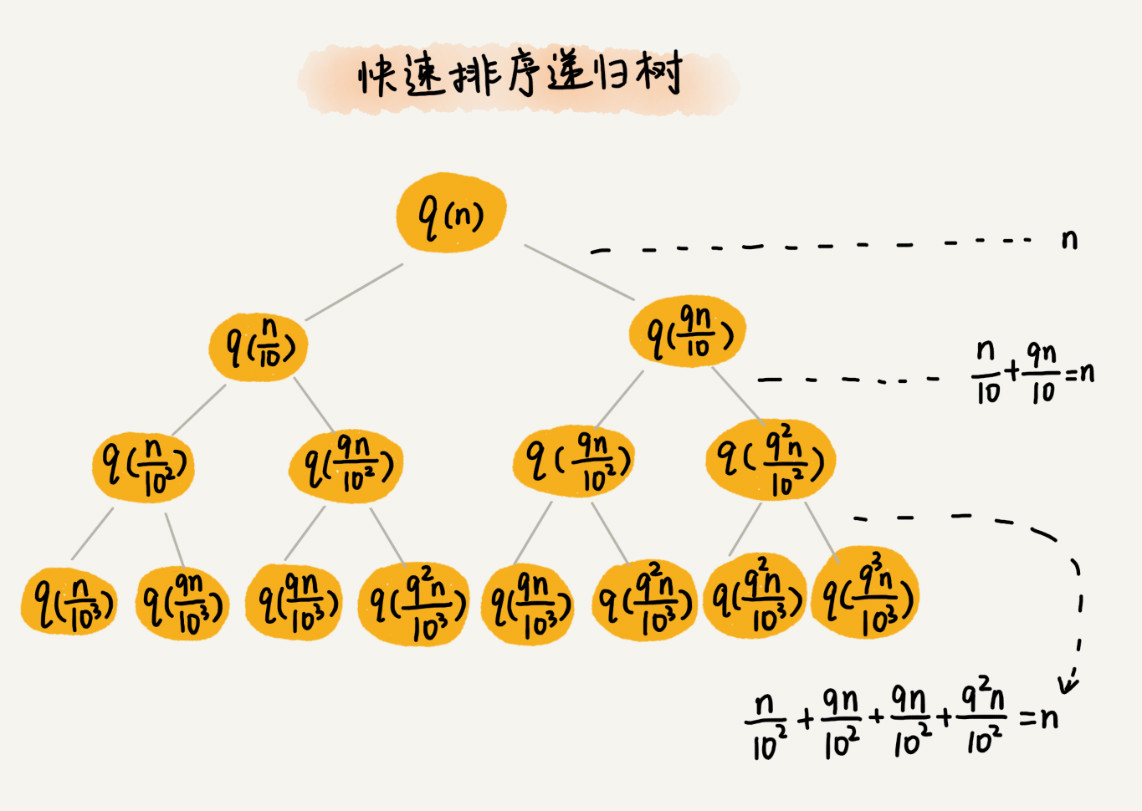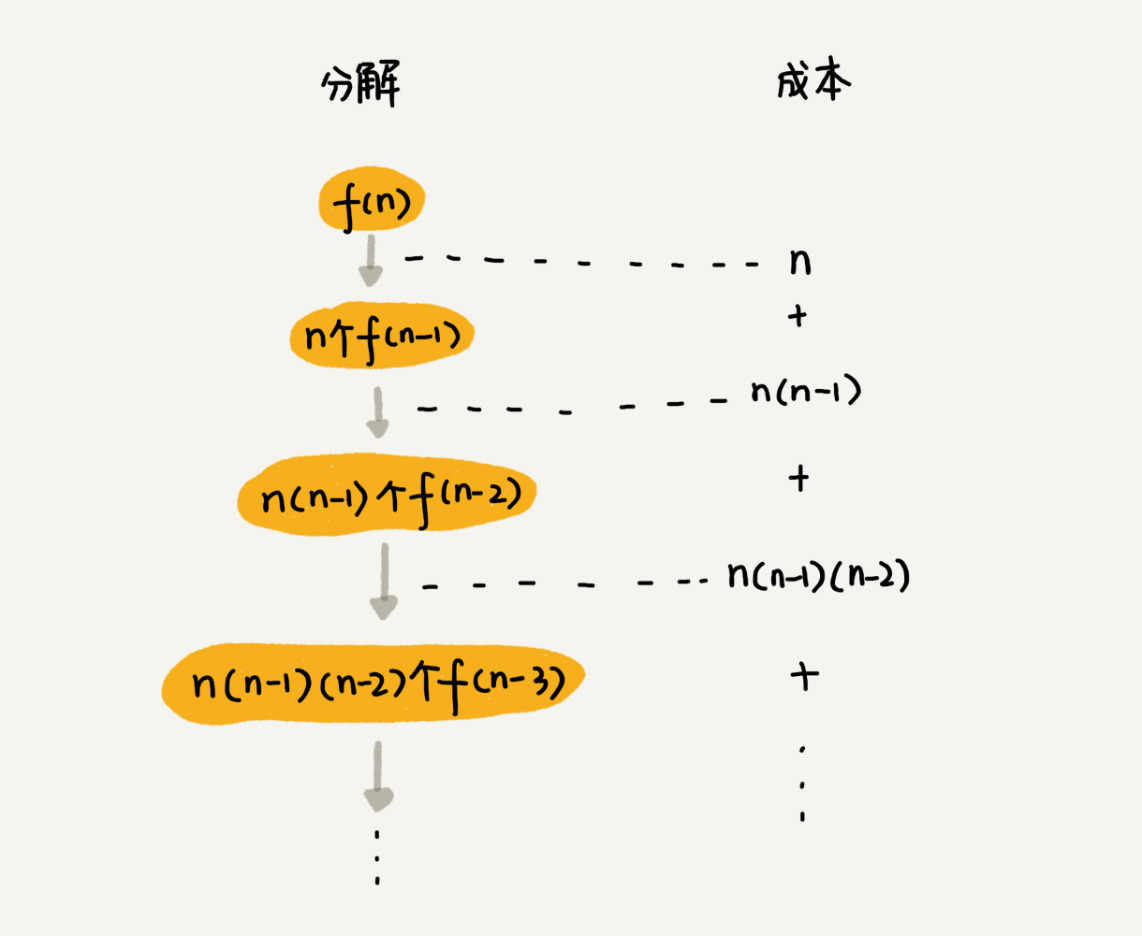## 递归树与时间复杂度分析## 实战一：分析快速排序的时间复杂度## 实战三：分析全排列的时间复杂度

假设数组中存储的是 1，2， 3...n。

f(1,2,...n) = {最后一位是 1, f(n-1)} + {最后一位是 2, f(n-1)} +...+{最后一位是 n, f(n-1)}。

// 调用方式：
// int[]a = a={1, 2, 3, 4}; printPermutations(a, 4, 4);
// k 表示要处理的子数组的数据个数
public void printPermutations(int[] data, int n, int k) {
if (k == 1) {
for (int i = 0; i < n; ++i) {
System.out.print(data[i] + " ");
}
System.out.println();
}

for (int i = 0; i < k; ++i) {
int tmp = data[i];
data[i] = data[k-1];
data[k-1] = tmp;

printPermutations(data, n, k - 1);

tmp = data[i];
data[i] = data[k-1];
data[k-1] = tmp;
}
}n + n*(n-1) + n*(n-1)*(n-2) +... + n*(n-1)*(n-2)*...*2*1

## 思考

• 1个细胞的生命周期是 3 小时，1 小时分裂一次。求 n 小时后，容器内有多少细胞？请用递归时间复杂度的分析方法，分析一下这个递归问题的时间复杂度。